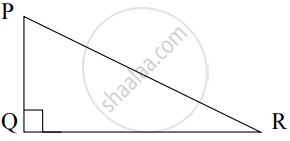# Complete the following activity to find the length of hypotenuse of right angled triangle, if sides of right angle are 9 cm and 12 cm. Activity: In ∆PQR, m∠PQR = 90°By Pythagoras Theorem, PQ2 + □ = P - Geometry

Sum

Complete the following activity to find the length of hypotenuse of right angled triangle, if sides of right angle are 9 cm and 12 cm.

Activity: In ∆PQR, m∠PQR = 90°By Pythagoras Theorem,

PQ2 + square = PR2    ......(I)

∴ PR2 = 92 + 122

∴ PR2 = square + 144

∴ PR2 = square

∴ PR = 15

∴ Length of hypotenuse of triangle PQR is square cm.

#### Solution

In ∆PQR, m∠PQR = 90°

By Pythagoras Theorem,

PQ2 + QR2 = PR    ......(I)

∴ PR2 = 92 + 122

∴ PR2 = 81 + 144

∴ PR2 = 225

∴ PR = 15      ......[Taking square roots of both sides]

∴ Length of hypotenuse of triangle PQR is 15 cm.

Concept: Similarity in Right Angled Triangles
Is there an error in this question or solution?

Share